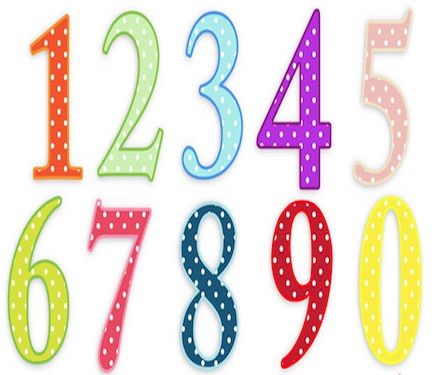# Number Sense# Tables

Although the Mathematical Reasoning module of the GED allows you to utilize a calculator for most of its questions, knowledge of mathematical tables is useful for the five
calculator-prohibited questions.

Knowing multiplication and division tables is particularly helpful with factoring and simplifying a square root.

Knowing multiplication and division tables is also helpful for working with equations and inequalities.

Knowing mathematical tables has a way of coming in handy in real life, too.

table_subtraction

table_multiplication

table_division

# Scale# Ratio and Proportion# Square Root

Square Root =  Number² = √ Number × Itself

# Factors and Multiples

Factor × Factor = Multiple

# SquaringNumber Squared = Number × Itself = Number²